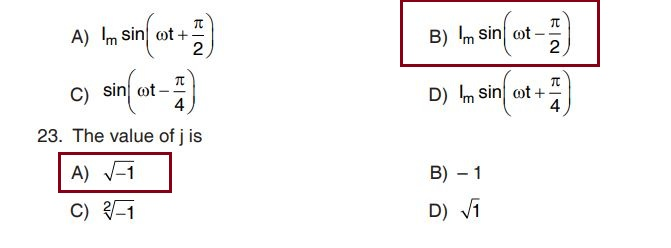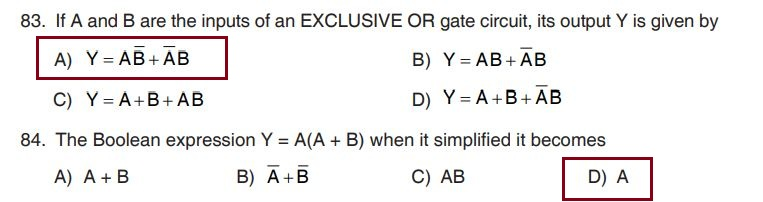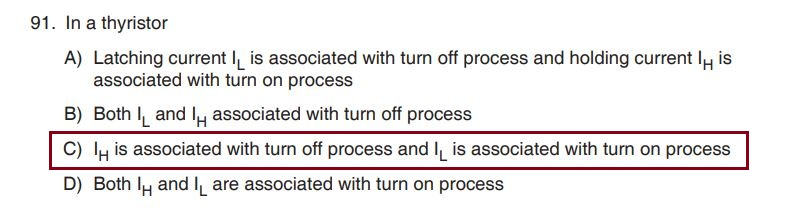3. Resistance of a material is independent of
A) Magnetic flux
B) Length
C) Nature of material
D) Temperature

4. What will be the current drawn by a lamp of rating 230 V, 100 W when it is connected across 50 V dc supply ?
A) 0.0945 A
B) 0.0261 A
C) 0.0831 A
D) 0.0362 A

9. Two resistors 4 Ohm and R Ohm are connected in parallel across 20 V supply. What will be the value of unknown resistor R when 100 W power is dissipated in it ?
A) 6
B) 4
C) 4.1
D) 6.2

10. The circuit whose properties or characteristics are the same in either direction is
A) Liner
B) Non liner
C) Both A) and B)
D) None of these14. If in shifting one Coulomb of positive charge from infinity to a certain point in the electric field, the work done and potential of that point are
A) One Joule and one volt
B) One Coulomb and one volt
C) One Coulomb and one newton
D) None of the above

15. The capacitance of multiplate capacitor is17. The ratio of intensity of magnetisation I to the magnetising force H is called
A) Flux density
B) Magnetic potential
C) Permeance
D) Susceptibility

18. Which parameter in magnetic circuit is analogous to resistivity in electric circuit ?
A) Permeance
B) Permeability
C) Reluctivity
D) Reluctance

19. Ratio of total flux to useful flux is
A) MMF
B) Retentively
C) Coercive force
D) Leakage coefficient

20. Which one is the Diamagnetic material ?
A) Aluminium
B) Nickel
C) Bismuth
D) Iron

21. Amplitude factor is the ratio of
A) Average value and Maximum value
B) RMS value and Average value
C) Maximum value and RMS value
D) Average value and Maximum value

22. The current flowing in a purely inductive circuit is24. Q factor is the ratio of
A) Z/R
B) R/Z
C) L/R
D) R/L

25. A coil is connected in series with a pure capacitor. The combination is fed from a 10 V supply of 10000 Hz. It was observed that the maximum current of 2 A flows in the circuit when the capacitor is of value 1 microfarad. What is the value of R ?
A) 10
B) 5
C) 6
D) 5.530. In a three phase star connected system the angle between line voltage and its phase voltage is
A) 90
B) 60
C) 30
D) 180

31. One of the following meter is not used for measuring a dc electric quantity
A) Voltmeter
B) Ammeter
C) Wattmeter
D) Power factor meter

32. One of the following instrument gives multiple electric quantities from a connected circuit
A) TOD meter
B) Insulation tester
C) Multimeter
D) Synchroscope

33. The easiest way to identify a PMMC instrument is
A) Uniform scale
B) Non uniform scale
C) Knife edge needle
D) Parallax mirror fitted

34. In two wattmeter method of three phase power measurement, when the power factor is 0.5 lagging
A) Both wattmeters will read equal power
B) One wattmeter will read total power
C) Readings of two wattmeters are equal and opposite
D) None of the above

35. The range of a voltmeter can be extended by
A) Connecting a high value resistor in series with the meter
B) Connecting a low value resistor is parallel the meter
C) Connecting a high value resistor is parallel the meter
D) Connecting a low value resistor in series the meter

36. A synchroscope is used for
A) Power factor measurements
B) Energy measurements
C) Frequency measurements
D) None of the above

37. Which of the following meter having the possibility of creeping error ?
A) Voltmeter
B) Power factor meter
C) Energy meter
D) Wattmeter

38. Which of the following is a step-up transformer ?
A) Distribution transformer
B) Potential transformer
C) Current transformer
D) None of the above

39. A CRO is not used for
A) Voltage measurement
B) Current measurement
C) Phase measurement
D) Frequency measurement

40. Which of the following can be used for inductance measurements ?
A) Maxwell bridge
B) Wheatstone bridge
C) Wein bridge
D) Schering bridge

41. Which of the following is an electrical safety requirement ?
A) Use IS specified materials for all installation
B) Common Fuse fitted for two or more sub circuit
C) All the circuit must be wire up with one square mm wire
D) The power circuit is connected through ELCB with 30mA rating in an industry

42. Which of the incorrect first aid provided to a person who have an electric hazard ?
A) Immediately give drinking water
B) Immediately remove the electrical contact from the body
C) Immediately give CPR if needed
D) Immediately bring into medical support

43. Which of the correct statement for an electrical installation ?
A) Fuse or switch is must be provided in earth conductor
B) No socket outlet is to be provided in the bathroom
C) A switch board is to be installed so that its bottom lies 1.25 meter above the floor
D) Don’t use armored cable for internal installation

44. Which one of the following is wrong for testing of insulation resistance between the wiring and earth ?
A) Main switch is in off position
B) Main fuse is taken out
C) All the switches are in off position
D) All other fuses are in position

45. In a lighting sub circuit, the total load/No. of points shall be limited to
A) 700 W/10 points
B) 800 W/10 points
C) 900 W/12 points
D) 1000 W/12 points

46. Which one of the following is an indication of fully charged 12 V battery ?
A) Terminal voltage is 12 V
B) Rise in temperature
C) Specific gravity of electrolyte is 1.21
D) None of the above

47. Which of the following is an electrolyte in lead acid battery ?
A) Dilute nitric acid
B) Dilute hydrochloric acid
C) Dilute sulfuric acid
D) Distilled water

48. The capacity of battery is expressed in
A) Watt hour
B) Kilo watt hour
C) Ampere hour
D) Volt ampere

49. Which type solar cell is more efficient ?
A) Poly crystal
B) Mono crystal
C) Thin film
D) None of the above

50. What number of cell required for get 24 V terminal voltage of a lead acid battery ?
A) 10
B) 5
C) 12
D) 24

51. One of the following is a temporary wiring
A) Loop wiring
B) Concealed wiring
C) Open conduit wiring
D) CTS or TRS wiring

52. Which type of wiring is not suitable where acid or alkalis are likely to be present ?
A) Case and capping wiring
B) Conduit wiring
C) Cleat wiring

53. The IS specification of wire used for domestic wiring is
A) 5A 240V PVC insulated wire
B) 1 sq.mm 1100V PVC insulated wire
C) 1 sq.mm 240V PVC insulated wire
D) 5A 440V PVC insulated wire

54. As per IS specification maximum current carrying capacity of 1.5 sq.mm copper wire is
A) 5A
B) 10A
C) 15A
D) 20A

55. The material used for fuse element is
A) Alloy of lead and copper
B) An alloy of tin and lead
C) An alloy of tin and aluminum
D) Thin copper conductor

56. The most commonly used fuse in house wiring is
A) HRC fuse
B) Drop holder fuse
C) Kit-kat fuse
D) Cartridge fuse

57. One of the material is not used as a filler in the HRC fuse
A) Calcium carbonate
B) Sand
C) Quartz
D) Graphite powder

58. The one of the switch is used as isolator of star delta starter
A) SPST
B) DPST
C) ICTP
D) ICDP

59. Standard size of copper plate for plate earthing is
A) 50 x 50 cm 6.3 mm
B) 60 x 60 cm 3.15 mm
C) 60 x 60 cm 6.3 mm
D) 50 x 50 cm 3.13 mm

60. MCB used for an electrical installation is
A) Electric shock protection
B) Over current protection
C) Over voltage protection
D) All of the above

61. In d.c. machine the yoke is made of
A) Cast steel
B) Soft iron
C) Silicon steel
D) None of the above

62. The armature of a d.c. machine is laminated in order to reduce
A) Copper loss
B) Eddy current loss
C) Hysteresis loss
D) Frictional loss

63. In d.c. machine the armature winding is placed on rotor to
A) Reduce the armature reaction
B) Facilitate commutation
C) Save iron
D) Reduce losses

64. In a d.c. machine, the number of armature segments is equal to
A) Number of conductors
B) Twice the number of poles
C) Number of coils
D) None of the above

65. The parallel paths of an 8 pole duplex lap winding will be
A) 4
B) 8
C) 16
D) 32

66. Carbon brushes are preferable to copper brushes due to
A) They have longer life
B) They reduce armature reaction
C) They have lower resistance
D) They reduce sparking

67. The speed of a d.c. motor can be controlled by
A) Applied voltage
B) Its flux
C) Armature circuit resistance
D) All of the above

68. Motor starters are essential for
A) Starting the motor
B) Accelerating the motor
C) Avoiding excessive starting current
D) Preventing fuse blowing

69. The efficiency of a d.c. motor when developing maximum mechanical power will be
A) Less than 50%
B) 50%
C) More than 50%
D) 100%

70. If the load current and magnetic flux of a d.c. motor are held constant and voltage applied across its armature is increased by 5%, the speed of the motor will
A) Remain unaltered
B) Decrease by 5%
C) Depend on other factors
D) Increase by 5%

71. The primary and secondary of a transformer are coupled
A) Electrically
B) Magnetically
C) Electrically and magnetically
D) None of the above

72. A two winding transformer operates at maximum efficiency when its
A) Hysteresis loss equal eddy current loss
B) Voltage regulation is minimum
C) Copper loss equals iron loss
D) Primary resistance equals secondary resistance

73. The transformer that does not provide electric isolation is
A) Power transformer
B) Potential transformer
C) Current transformer
D) Auto transformer

74. The synchronous speed of a three phase induction motor having 20 poles and connected to a 50 Hz source is
A) 300 r.p.m.
B) 600 r.p.m.
C) 1000 r.p.m.
D) 1200 r.p.m.

75. In a squirrel cage induction motor, the maximum torque developed under running condition is
A) Equal to starting torque
C) Much higher than full load torque
D) Less than starting torque

76. The purpose of starting winding in a single phase induction motor is to
A) Produce rotating flux in conjunction with main winding
B) Reduce losses
C) Limit temperature rise of the machine
D) None of the above

77. The stator of an alternator is identical to that of a
A) d.c. generator
B) three phase induction motor
C) single phase induction motor
D) none of the above

78. An under excited alternator supplies
A) Lagging VAR
B) No reactive power
D) None of the above

79. Damper windings are used in alternators to
A) Prevent hunting
B) Reduce winding losses
C) Achieve synchronism
D) None of the above

80. A resistance split phase induction motor is used for
D) None of the above82. Nibble is
A) A string of 4 bits
B) A string of 8 bits
C) A string of 16 bits
D) A string of 64 bits85. The minimum number of flip-flop required to build a mode 20 counter is
A) 2
B) 3
C) 4
D) 5

86. The fan out of a logic gate is
A) The number of subsequent circuits which the gate can drive
B) The number of inputs connected to the gate without any degradation of voltage level
C) Number of connection to the package
D) None of the above

87. How many 7483 IC’s are required to add two bytes data ?
A) 1
B) 2
C) 3
D) 4

88. By placing an inverter to the input of an SR flip-flop, the resulting flip-flop becomes
A) JK flip-flop
B) D flip-flop
C) T flip-flop
D) M/S JK flip-flop

89. The number of flip-flop needed to divide input frequency by 64 is
A) 8
B) 16
C) 4
D) 6

90. Which of the following is not a specification of a D/A or A/D converter ?
A) Gain
B) Drift
C) Speed
D) Accuracy92. For an UJT employed for triggering of an SCR, Intrinsic strand off ratio n = 0.64 and dc source voltage = 20 V. The UJT would trigger when the emitter voltage is
A) 12.8 V
B) 13.5 V
C) 10 V
D) 5 V

93. In a properly biased JFET will acts as a
A) Current controlled current source
B) Voltage controlled voltage source
C) Voltage controlled current source
D) Current controlled voltage source

94. The structure of IGBT is a
A) N-N-P-P structure connected by a MOS gate
B) PNP structure connected by a MOS gate
C) PNPN structure connected by a MOS gate
D) N-P-N-P structure connected by a MOS gate

95. The anode current through a conducting SCR is 10 A, if its gate current is made one-fourth, then what will be the anode current ?
A) 0 A
B) 5 A
C) 10 A
D) 20 A

96. Which of the following device is used for triggering of TRIAC ?
A) FET
B) IGBT
C) DIAC
D) SCR

97. A boost regulator has an input voltage of 5 V and the average output of 15 V. The duty cycle is
A) 3/2
B) 2/3
C) 5/2
D) 15/299. Which one is used to protect a thyristor from di/dt condition ?
A) Inductor
B) Snubber circuit
C) Fuse
D) Zener diode

100. 6Ah in an UPS battery specification means
A) 6 A for one hour
B) 1 A for six hours
C) The output power is 6 w
D) It requires 5 hours to charge fully